# 2. 单自由度系统

《振动：解析与试验模态分析》 辛辛那提大学 Randall J. Allemang教授 (著)，KSI科尚仪器 董书伟 (译)

### 2.1 理论

$M \ddot{x}(t)+C \dot{x}(t)+K x(t)= f(t) \qquad \qquad \qquad (2.1)$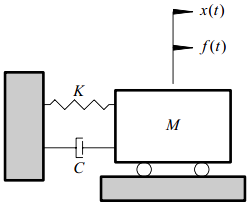$x(t)=x_{c}(t)+x_{p}(t)$

• $x_{c}(t)\;-\;$瞬态部分
• $x_{p}(t)\;-\;$稳态部分

$M \ddot{x}(t)+C \dot{x}(t)+K x(t)= 0 \qquad \qquad \qquad (2.2)$

$(Ms^2+Cs+k)X(s)e^{st}=0$

$s^{2}+\left ( C/M \right )s+\left ( K/M \right )=0 \qquad \qquad \qquad (2.3)$

$\lambda _{1,2}=-\dfrac{C}{2M}\pm \sqrt{\left ( \dfrac{C}{2M} \right )^{2}-\left ( \dfrac{K}{M} \right )}$

$x_{c}(t)=X_{1}e^{\lambda_{1}t}+X_{2}e^{\lambda_{2}t}$

### 2.2 拉氏域理论

$\begin{matrix} \mathbf{L}\left \{ M \ddot{x}+C \dot{x}+Kx \right \}=M\big( s^{2}X ( s ) -s \,x ( 0 )-\dot{x}( 0 ) \big) \\ \qquad \qquad \qquad +C \big( s\, X(s) -x(0) \big)+K\,X(s) \end{matrix}$

$\mathbf{L}\left \{ M \ddot{x}+C \dot{x}+Kx \right \}=(M\,s^2+C\,s+K)X(s)-M\,s\,x(0)-M\,\dot{x}(0)-C\,x(0)$

$\mathbf{L}\left \{ f(t) \right \}=F(s)$

$[M \, s^{2}+C \, s+K] \,X(s)=F(s)+(M\,s+C)\, x(0)+M \dot{x}(0) \qquad (2.4)$

• $x(0)$$t=0$时刻的初始位移
• $\dot{x}(0)$$t=0$时刻的初始速度

$[M \,s^2+C\,s+K]\,X(s)=F(s) \qquad \qquad (2.5)$

$B(s)=M\,s^2+C\,s+K$。称$B(s)$为系统阻抗。则式2.5变为：
$B(s)\,X(s)=F(s) \qquad \qquad (2.6)$

• $F(s)\,-$激振力函数$f(t)$的拉氏域（复频率）表示
• $X(s)\,-$系统响应$x(t)$的拉氏域（复频率）表示

$B(s)=\dfrac{F(s)} {X(s)}$

$X(s)=\dfrac{F(s)} {B(s)}\qquad \quad (2.7)$

$X(s)=H(s)\,F(s)\qquad \quad (2.8)$

$H(s)$为系统传递函数。换句话讲，传递函数建立了系统输入的拉氏变换与系统响应的拉氏变换之间的关系。根据式2.5和2.8，可以定义传递函数为：
$H\left ( s \right )=\dfrac{X(s)}{F(s)}=\dfrac{1/M}{s^2+(C/M)\,s+(K/M)} \qquad \quad (2.9)$

$\lambda _{1,2}=-(C/2M) \pm \sqrt{(C/2M)^2-(K/M)} \qquad \quad (2.10)$

### 2.3 名词解释

#### 2.3.1 临界阻尼

$(C_{c}/2M)^2-(K/M)=0$

$(C_{c}/2M)= \sqrt{K/M}=\Omega_{1}$

$C_{c}=2\,M \Omega_{1}= \;$临界阻尼系数

$\Omega_{1}= \;$无阻尼固有频率$(rad/sec)$

#### 2.3.2 临界阻尼分数 – 阻尼比（$\zeta$）

$\zeta_{1}=\dfrac{C}{C_{c}}$

$\lambda _{1,2}=(- \zeta_{1} \pm \sqrt{\zeta_{1}^{2}-1}) \Omega _{1} \qquad (2.11a)$

$\lambda _{1,2}=(- \zeta_{1} \pm j\sqrt{1 - \zeta_{1}^{2}}) \Omega _{1} \qquad (2.11b)$

### 2.4 系统分类

• 过阻尼系统：$\quad \zeta _{1} > 1$
• 临界阻尼系统：$\, \zeta _{1} = 1$
• 欠阻尼系统：$\quad \zeta _{1} \lt 1$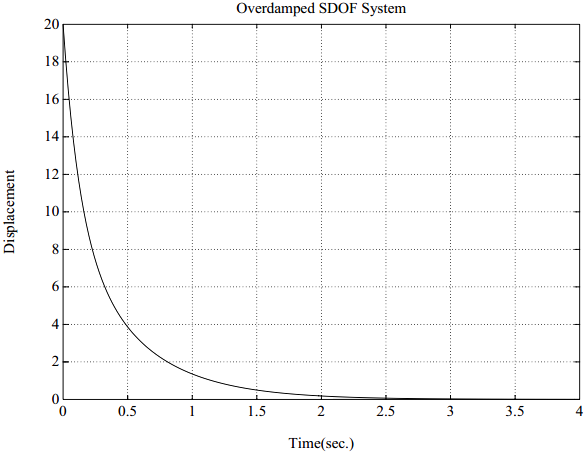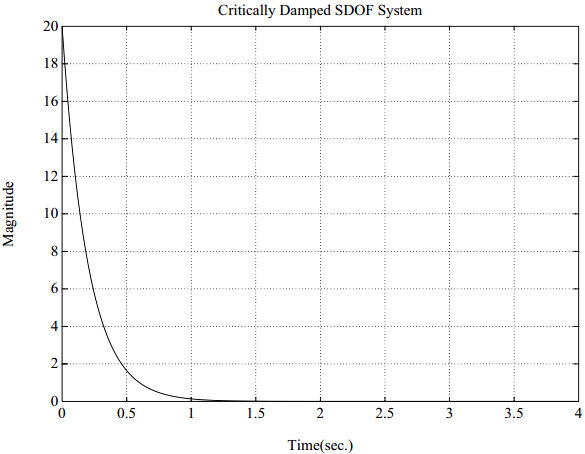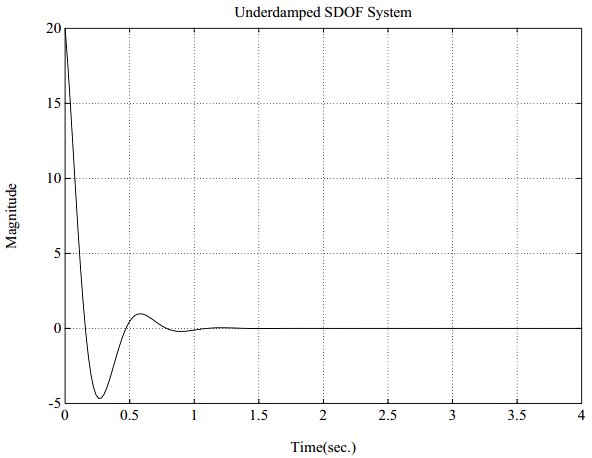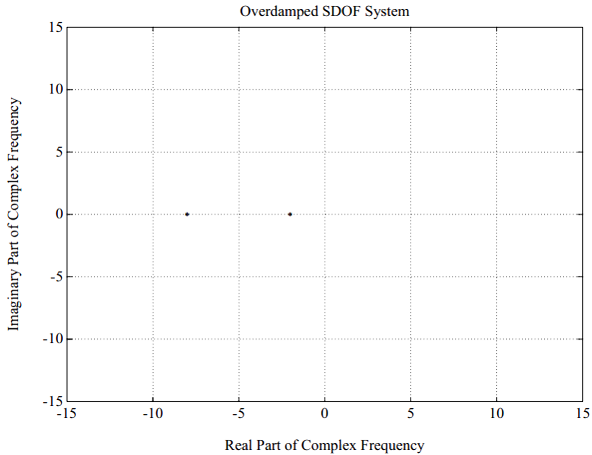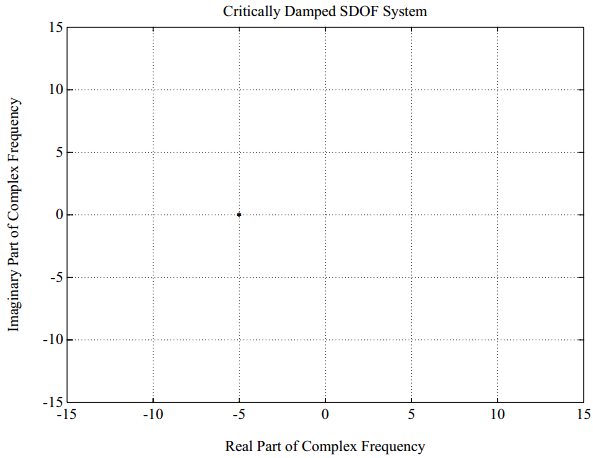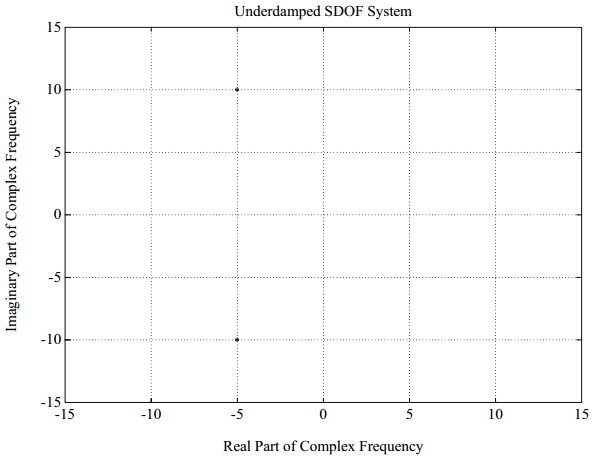$\lambda _{1,2}=\sigma _{1} \pm j \omega _{1}$

• $\sigma _{1}$为阻尼因子（单位$rad/sec$
• $\omega _{1}$为阻尼固有频率

\begin{aligned} \zeta _{1} &= -\dfrac{\sigma _{1}}{\sqrt{\omega_{1}^{2}+\sigma _{1}^{2}}} \\ \sigma _{1} &= - \zeta _{1} \Omega _{1} \\ \Omega _{1} &= \sqrt{\omega_{1}^{2}+\sigma _{1}^{2}} \end{aligned}

$H(s)=\dfrac{1/M}{(s-\lambda _{1})(s- \lambda_{1}^{*})} \qquad \qquad (2.12)$

• $\lambda \;\,=\;$传递函数的极点
• $\lambda _{1} = \sigma _{1}+j \omega _{1}$
• $\lambda _{1}^{*} = \sigma _{1} - j \omega _{1}$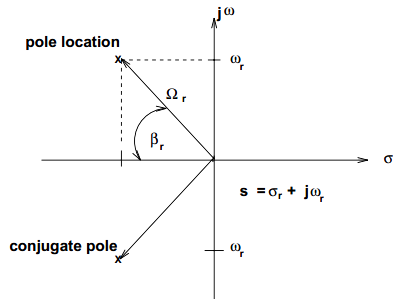• $\sigma _{r} - \;$阻尼系数
• $\omega _{r} - \;$阻尼固有频率
• $\Omega _{r} - \;$共振频率（无阻尼固有频率）
• $\zeta _{r}=\cos \beta _{r} - \;$阻尼因子（或称为临界阻尼百分比）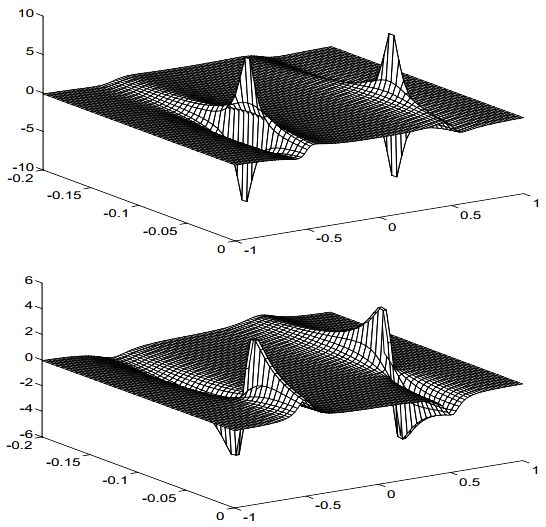### 2.5 解析模型-标量多项式

$H(s)= \dfrac{ \beta _{0}}{ \alpha_{2}(s)^{2}+ \alpha_{1}(s)^{1}+ \alpha _{0}(s)^{0}} \qquad \qquad (2.13)$

$H(s)= \dfrac{ \beta _{0}}{ \displaystyle\sum _{k=0}^{2} \alpha _{k}(s)^{k} } \qquad \qquad (2.14)$

### 2.6 解析模型-部分分式

$H(s)=\dfrac{1/M}{(s-\lambda _1)(s-\lambda _{1}^*)}=\dfrac{c_1}{(s-\lambda 1)}+\dfrac{c_2}{(s-\lambda _{1}^{*})} \qquad \qquad (2.15)$

$\dfrac{1/M}{(s-\lambda _{1}^{*})}|_{s= \lambda _1}=c_{1}+\left [ \dfrac{c_2(s- \lambda_1)}{(s- \lambda_{1}^*)} \right ]|_{s= \lambda_1}$

$\dfrac{1/M}{(\lambda _{1}-\lambda _{1}^{*})}=c_{1}$

$c_{1}=\dfrac{1/M}{(\sigma _{1}+j \omega_{1})-(\sigma _{1}-j \omega_1)}= \dfrac{1/M}{j\,2\, \omega_1}=A_1$

$c_2 = \dfrac{1/M}{-j \,2\,\omega_1}=A_{1}^{*}$

$H(s)=\dfrac{A_1}{(s-\lambda _1)}+\dfrac{A_{1}^{*}}{(s-\lambda _{1}^*)} \qquad \qquad (2.16)$

### 2.7 频响函数

$H(s)|_{s= j \omega}=H(\omega)= \dfrac{ \beta _0}{\alpha_{2}(j \omega)^{2}+ \alpha_{1}(j \omega)^{1}+ \alpha_{0}(j \omega)^0} \qquad \qquad (2.17a)$

\begin{aligned} H(s)|_{s=j \omega} &= H(\omega) =\dfrac{A_1}{(j \omega - \lambda_1)}+\dfrac{A_{1}^*}{(j \omega - \lambda_{1}^*)} \\ H(\omega) &=\dfrac{A_1}{(j \omega - \sigma_{1} - j \omega_1)}+\dfrac{A_{1}^*}{(j \omega - \sigma_{1}+j \omega_1)} \\ H(\omega) &=\dfrac{A_1}{j (\omega - \omega_1) -\sigma_{1}}+\dfrac{A_{1}^*}{j (\omega + \omega_1)- \sigma_{1}} \end{aligned} \qquad \quad \qquad (2.17b)

$H(\omega_1)=-\dfrac{A_{1}}{\sigma _1}+\dfrac{A_{1}^*}{j\,2\,\omega_{1}-\sigma_1} \qquad \qquad (2.18)$

$H(\omega_1)=-\dfrac{A_{1}}{\sigma _1}$

$\omega_1$增大时，式2.18右侧的第2项趋向于零。换句话说，频响函数负频率部分的贡献可以忽略不计。

$H(\omega)\approx -\dfrac{A_{1}}{\left (j \omega - \lambda_{1} \right )} \qquad \qquad (2.19)$

### 2.8 脉冲响应函数表达式

$X(s) =\dfrac{A_1}{(s-\lambda _1)}+\dfrac{A_{1}^*}{(s-\lambda _{1}^*)}$

$x(t)=\mathbf{L}^{-1}\left \{ X(s) \right \}$

$x(t)=A_{1}e^{\lambda_{1}t}+A_{1}^{*}e^{\lambda_{1}^{*}{t}}=h(t)=\,$脉冲响应

$x(t)=e^{\sigma_{1}t}\left [ A_{1}e^{j\omega_{1}t}+A_{1}^{*}e^{-j\omega_{1}t} \right ]$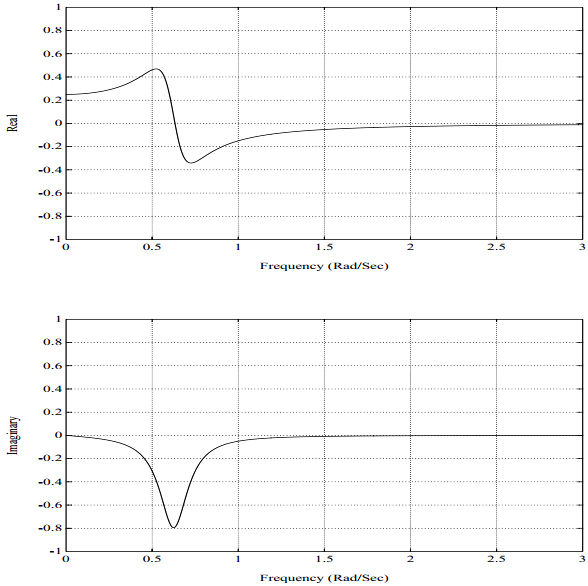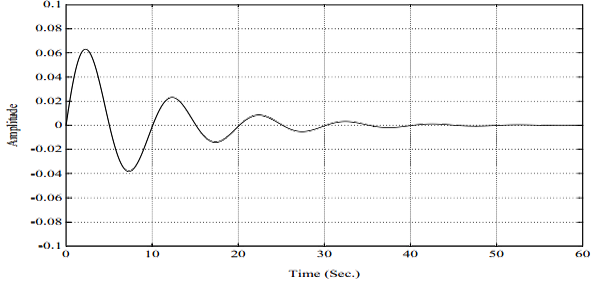### 2.9 物理参数变化对频响函数的影响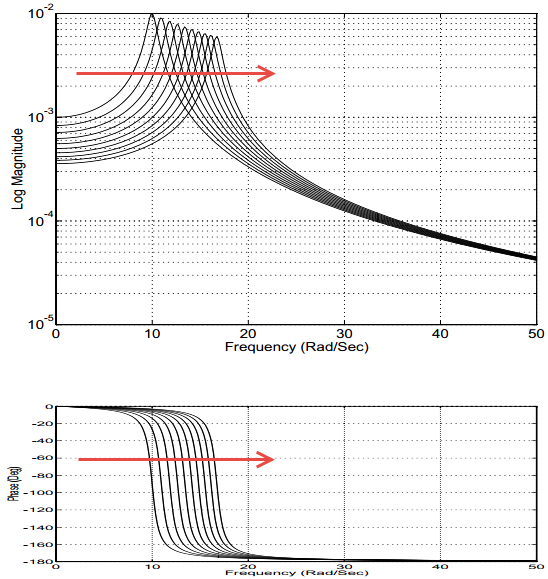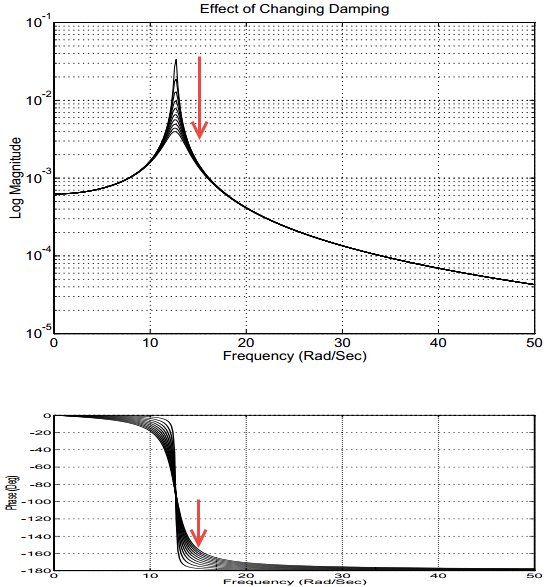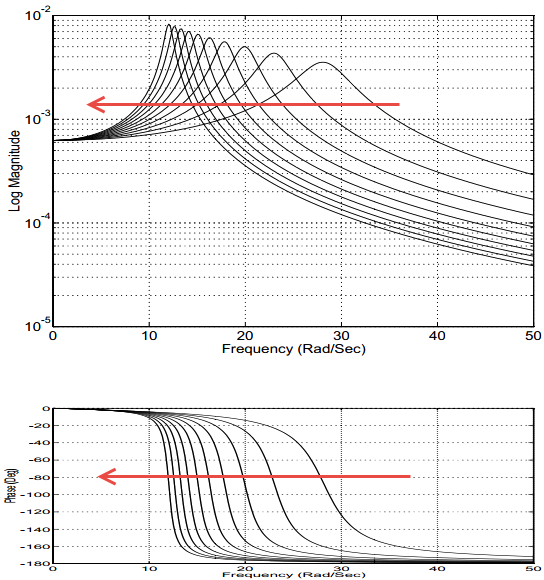### 2.10 估计部分分式参数

$H(j \omega)=\dfrac{A_1}{(j \omega- \lambda_1)}+ \dfrac{A_{1}^{*}}{(j \omega - \lambda_{1}^{*})} \qquad \qquad (2.20)$

• 频响函数幅值达到最大值处对应的频率
• 频响函数实部过零处对应的频率
• 频响函数虚部达到相对极小值（或极大值）处对应的频率

$\zeta_{1}=\dfrac{\omega_{b}^{2}-\omega_{a}^{2}}{(2\,\omega_1)^2} \qquad \qquad (2.21)$

$\zeta_{1} \approx \dfrac{\omega _{b}- \omega _{a}}{2\, \omega_1} \qquad \qquad (2.22)$

$\sigma_{1}=- \zeta_{1} \Omega_{1} \qquad \;\qquad (2.23)$

$\sigma_{1} \approx -\zeta_{1} \omega_{1} \qquad \; \qquad (2.24)$

$H(\omega _1)=\dfrac{A_1}{j \omega_{1}-(\sigma_{1}+j \omega_{1})}+\dfrac{A_{1}^{*}}{j \omega_{1}-(\sigma_{1}-j \omega_{1})} \qquad \qquad\ (2.25)$

$H(\omega _1)=\dfrac{A_1}{(-\sigma_1)}+\dfrac{A_{1}^{*}}{(2\,j \omega_{1}-\sigma_{1})} \qquad \qquad (2.26)$

$H(\omega _1) \approx \dfrac{A_1}{(-\sigma_1)} \qquad \qquad\ (2.27)$

$A_{1} \approx (- \sigma _{1})H( \omega _{1}) \qquad \qquad\ (2.28)$#### 2.10.1 示例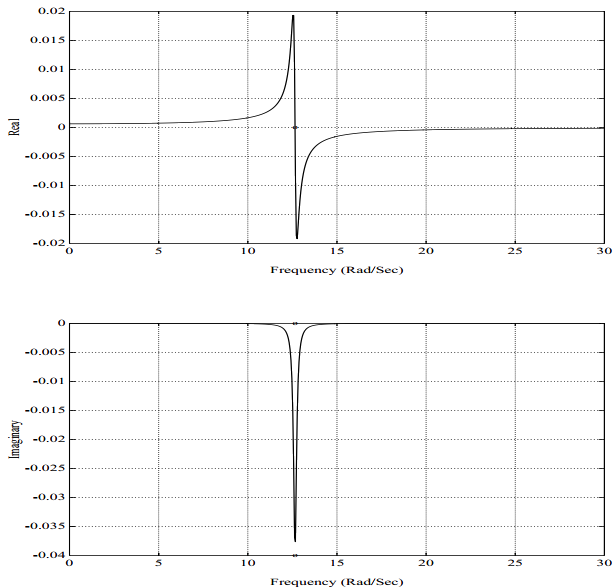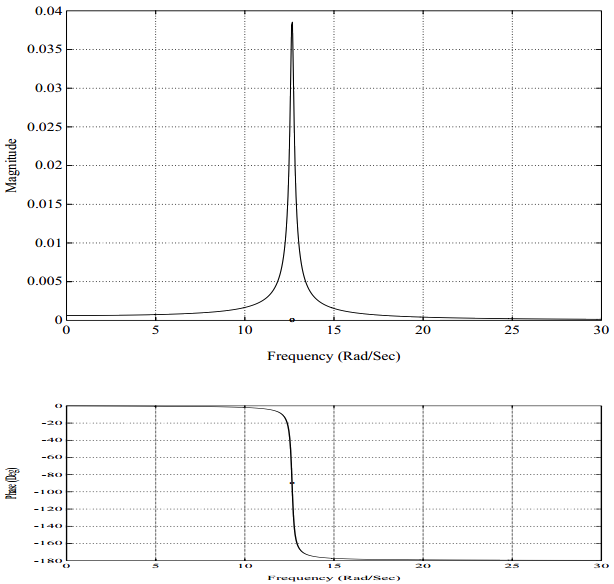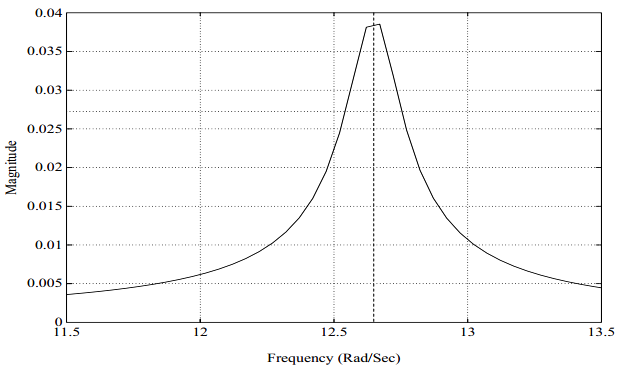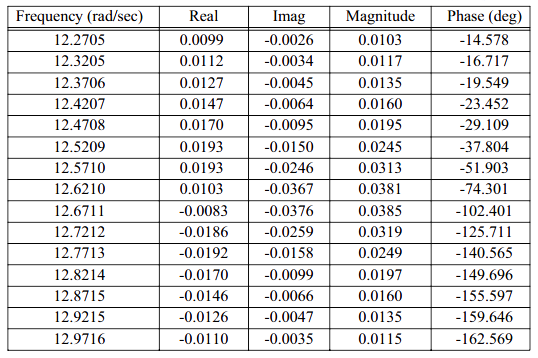### 2.11 为何要学习/强调单自由度系统？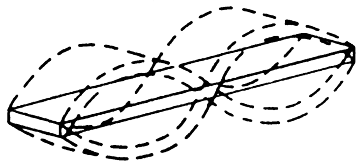$M \ddot{x}(t)+C \dot{x}(t)+K x(t)=f(t) \qquad \qquad (2-29)$

$f(t)=\displaystyle\sum_{\omega =0}^{\infty}F(\omega)e^{j \omega t} \qquad \qquad (2-30)$

$x(t)=\displaystyle\sum_{\omega =0}^{\infty}X(\omega)e^{j \omega t} \qquad \qquad (2-31)$

$H(\omega)=\dfrac{X(\omega)}{F(\omega)}=\dfrac{1}{-M \omega ^{2}+C\,j \omega+K} \qquad \qquad (2-32)$

$H_{qp}(\omega)=\dfrac{X_{q}(\omega)}{F_{p}(\omega)}=\dfrac{1}{-M \omega ^{2}+C\,j \omega+K} \qquad \qquad (2-33)$

$H_{qp}(\omega)=\dfrac{X_{q}(\omega)}{F_{p}(\omega)}=\dfrac{\psi_{q} \psi_{p}}{-M \omega ^{2}+C\,j \omega+K} \qquad \qquad (2-34)$

$H_{qp}(\omega)= \dfrac{X_{q}(\omega)}{F_{p}(\omega)}=\displaystyle\sum_{r =1}^{\infty}\dfrac{\psi_{qr} \psi_{pr}}{-M_{r} \omega ^{2}+C _{r}\,j \omega+K _{r}} \quad \qquad (2-35)$

$H_{qp}(\omega)= \dfrac{X_{q}(\omega)}{F_{p}(\omega)} \approx \dfrac{\psi_{qr} \psi_{pr}}{-M_{r} \omega ^{2}+C _{r}\,j \omega+K _{r}} \quad \qquad (2-36)$

$\alpha _{r} \approx \dfrac{\psi_{pr}}{-M_{r} \omega ^{2}+C _{r}\,j \omega+K _{r}} \quad \qquad (2-37)$

${x(t_i)}=\displaystyle\sum_{r =1}^{N}\beta _{r}\left \{ \psi _r \right \} \quad \qquad (2-38)$

${X(\omega_{i})}=\displaystyle\sum_{r =1}^{N}\beta _{r}\left \{ \psi _r \right \} \quad \qquad (2-39)$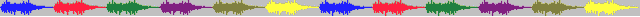《模态空间系列文章》Peter Avitabile教授 (著) KSI科尚仪器 董书伟 (译)…

《振动：试验模态分析》辛辛那提大学 Randall J. Allemang教授 (著) KSI科尚仪器 董书伟 (译)…[new]